# Constructing a Pentagon (Inscribed in a Circle)

Compass and straight edge constructions are of interest to mathematicians, not only in the field of geometry, but also in algebra. For thousands of years, beginning with the Ancient Babylonians, mathematicians were interested in the problem of "squaring the circle" (drawing a square with the same area as a circle) using a straight edge and compass. This problem is equivalent to finding the area of a circle. It turns out that this is impossible, but no-one managed to prove this until 1882!

However, it is possible to construct a regular pentagon, inscribed in a circle.

For this construction, you will need a straight edge (ruler - but you won't be measuring anything), a pair of compasses, a pencil and paper. I have drawn the pictures using the robocompass app. It's fun to play with, and you can use it to do all sorts of geometric constructions. There's a little bit of coding to learn, but a list of instructions is provided. Once you've written your little program, you can invite a few friends over to enjoy the beauty of the construction. This is probably the most complicated straight edge and compass construction on this site, so try to get a bit of experience with these constructions before you attempt this one. Be warned that, when you are using it to draw arcs, the robocompass compass point may appear to be a little away from the centre, but the drawing is actually accurate.

Note: In the interest of clarity, this construction assumes that you are familiar with constructing the perpendicular bisector of a line segment and constructing a perpendicular to a line segment at a point. If you're a bit hazy, I suggest you read the articles, and review these constructions first.

## The ConstructionStep 1: Start with a circle with its centre $O$ marked. If you don't know where the centre is, don't guess! Either draw a new circle, or use the construction for finding the centre of the circle.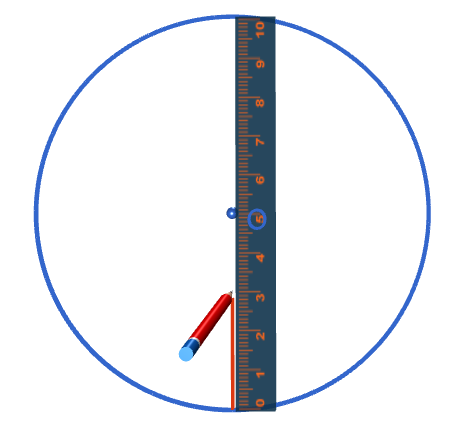Step 2: Use your straight edge to draw a diameter (a line through the centre of the circle, meeting it at two points) to the circle. Label the end points with $A$ and $I$. Point $I$ is going to be one of the vertices of your pentagon.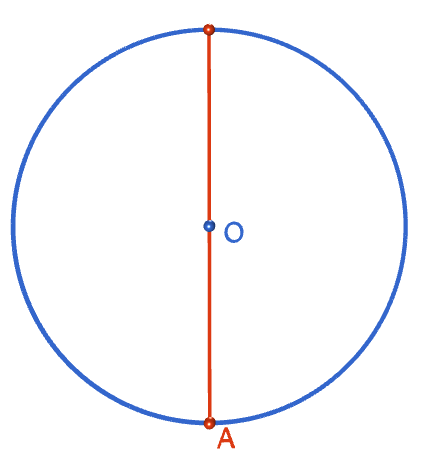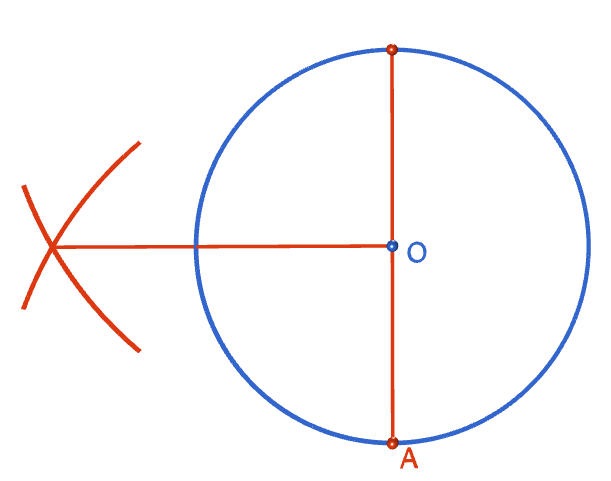Step 3: Construct the perpendicular to line segment $AI$ at the point $O$. Label the point where it cuts the circle with a $C$.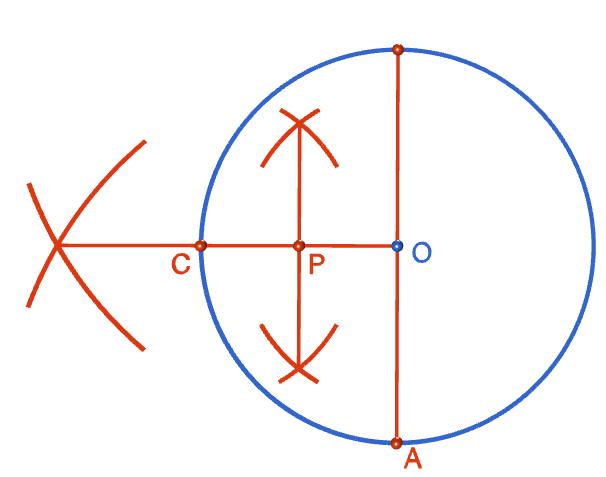Step 4: Construct the perpendicular bisector of line segment $OC$. Label the point where the bisector intersects the line segment with a $P$.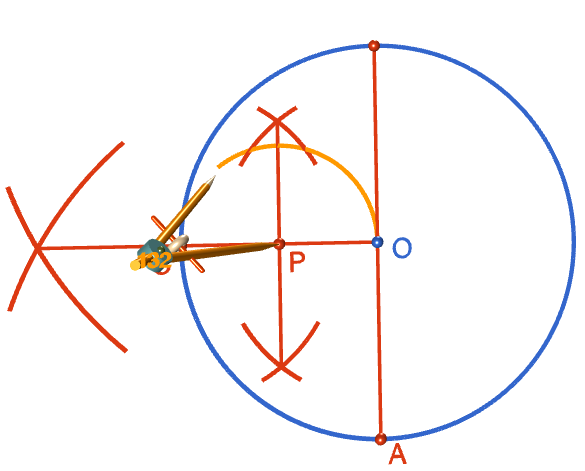Step 5: Open your compasses to a radius that matches the distance from point $O$ to point $P$. With the tip of your compasses on point $P$, draw a circle.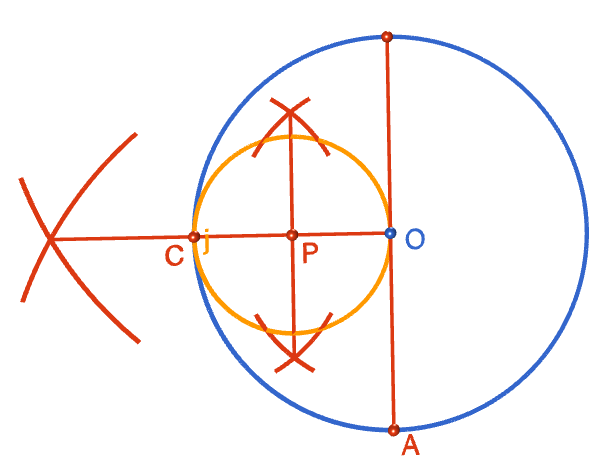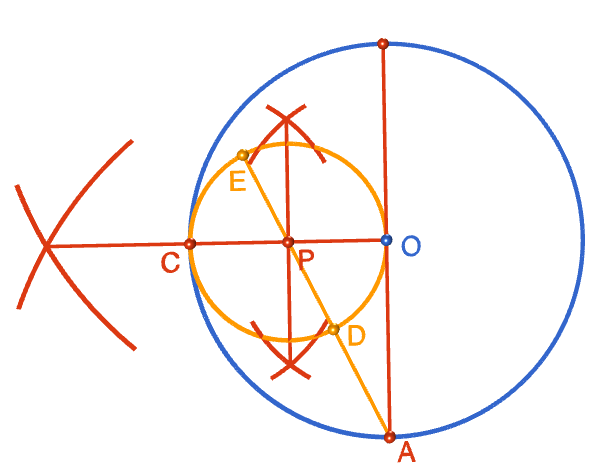Step 6: Using your straight edge, draw a line segment from point $A$, through point $P$, that cuts the small circle at two points. Label these points with a $D$ and an $E$. I've labelled them so that the $D$ is the closer one to point $A$.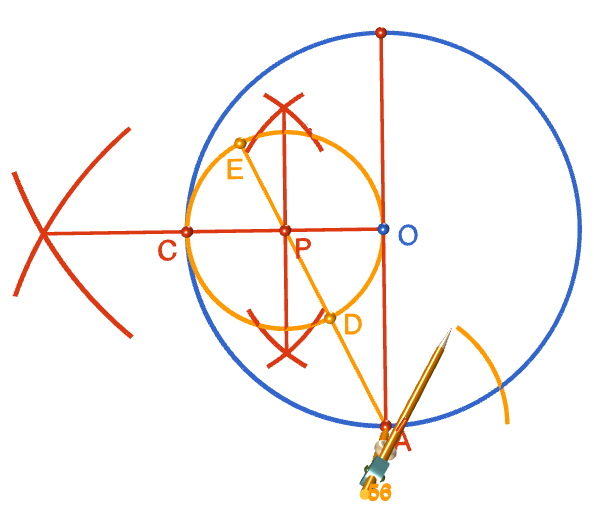Step 7: Put the tip of the compasses at point $A$, and open them to a radius that matches the distance from $A$ to $D$. Draw a (really big) arc that cuts the big circle in two places. Label those two points with $F$ and $G$. They are going to be two vertices of your pentagon.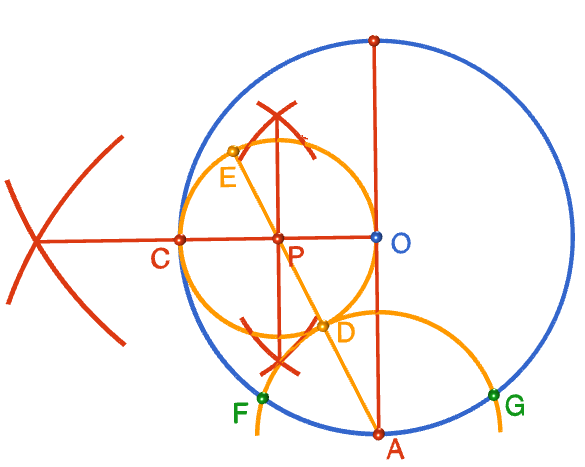Step 8: Would you believe we're almost there? Just this step, and then we can draw the edges. With the tip of your compasses at point $A$, open them out to a radius that matches the distances from $A$ to $E$ and draw an arc that cuts the big circle in two points. These will be the two remaining vertices of our pentagon. Label them with an $H$ and a $J$ as shown in the diagram.Step 9: It's time to join the dots. With your straight edge, rule line segments from $F$ to $G$, from $G$ to $H$, from $H$ to $I$, from $I$ to $J$ and from $J$ to $F$. Phew, it's done! Collapse in a heap on the floor, and then sit back to admire your help. Well done!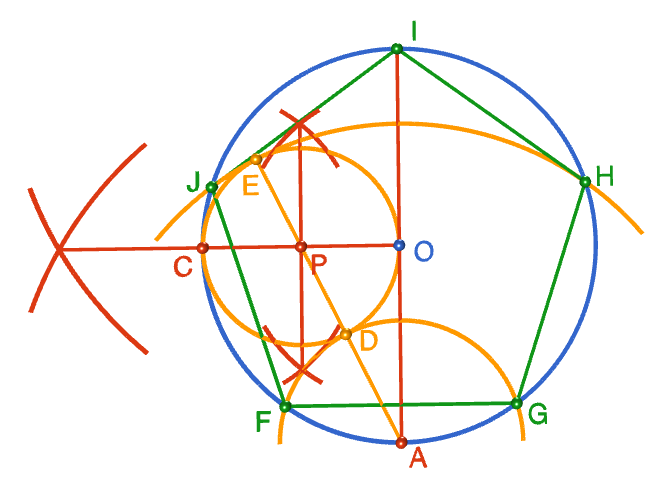### Description

This tutorial will expose you to Rulers and how to use them. We will also have a close look as set square and compass constructions. Your feedback is important to us, if you like any other topic covered under this tutorial, please do let us know.

### Audience

Year 10 or higher

### Learning Objectives

Ruler and compass constructions and more

Author: Subject Coach
You must be logged in as Student to ask a Question.